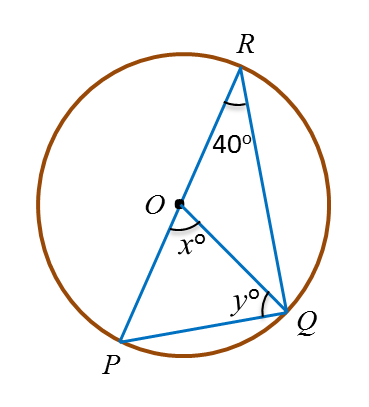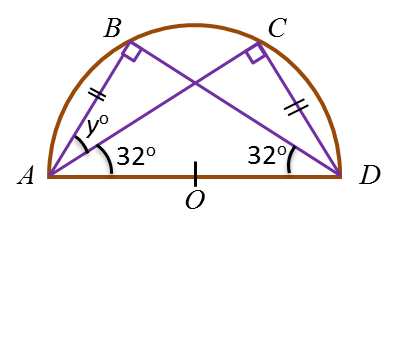# 6.6.2 Angles and Tangents of Circles, PT3 Practice

6.6.2 Angles and Tangents of Circles, PT3 Practice

Question 4:
Diagram below shows a circle with centre O. POR is a straight line.Find the value of x and of y.

Solution:
$\begin{array}{l}x=40×2\\ \text{}=80\\ \\ y=\frac{180-80}{2}\\ \text{}=50\end{array}$

Question 5:
Diagram below shows a semicircle ABCD with centre O.It is given that AB = CD.
Find the value of y.

Solution:y+ 32o + 32o + 90o = 180o
y= 180o – 154o
y= 26o
= 26

Question 6:
In diagram below, ABC and DEF are straight lines.Find the value of x and of y.

Solution: